# Selina Solutions Concise Maths Class 7 Chapter 2: Rational Numbers Exercise 2B

Selina Solutions Concise Maths Class 7 Chapter 2 Rational Numbers Exercise 2B helps students understand the steps to be followed in the representation of rational numbers on the number line and comparing rational numbers. To help students score well in the annual exam, experts at BYJU’S have created exercise wise problems. Students can download the PDF of solutions based on their requirements. They can solve the exercise wise problems without any time constraint. These solutions help students clear their confusion about the concepts discussed here. Selina Solutions Concise Maths Class 7 Chapter 2 Rational Numbers Exercise 2B PDF, links are given below.

## Selina Solutions Concise Maths Class 7 Chapter 2: Rational Numbers Exercise 2B Download PDF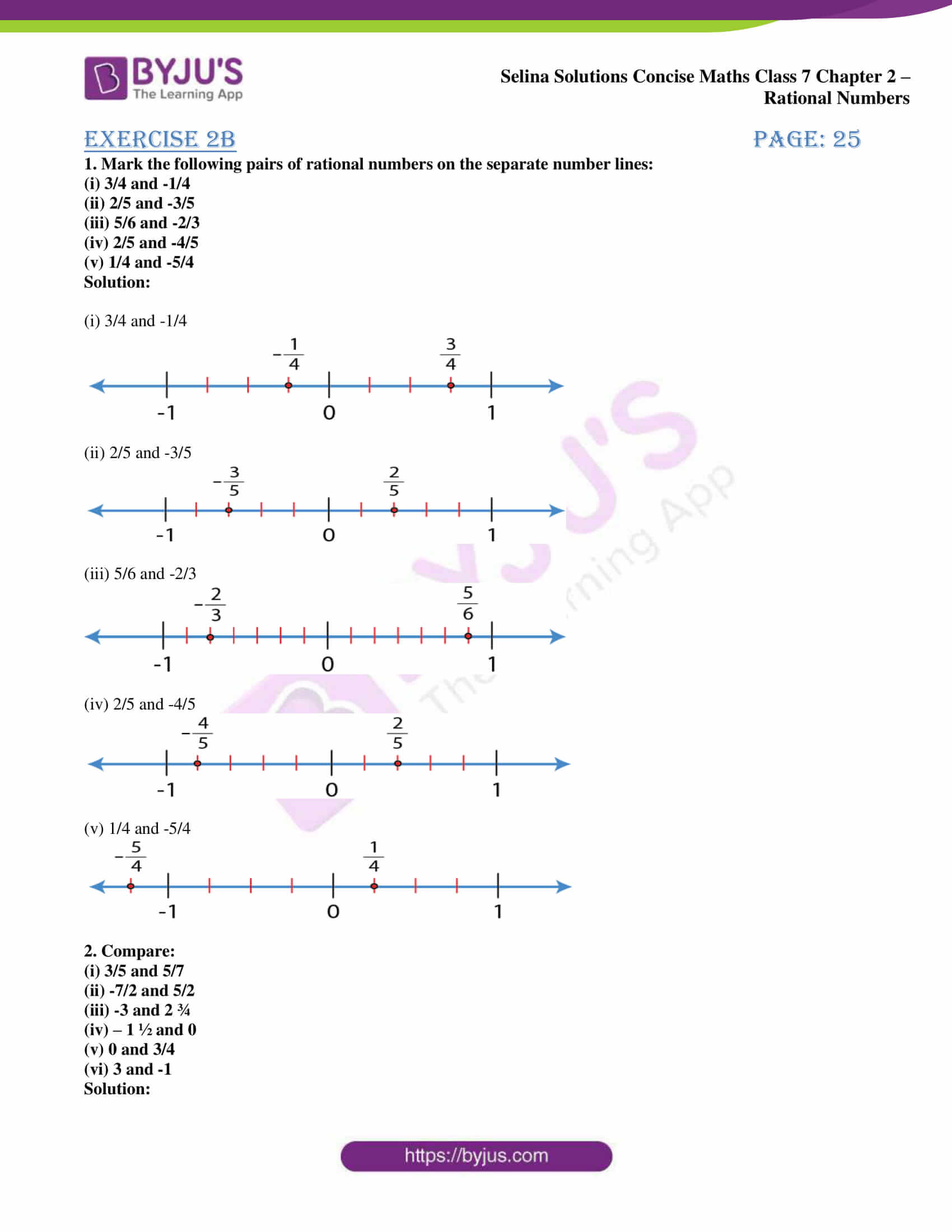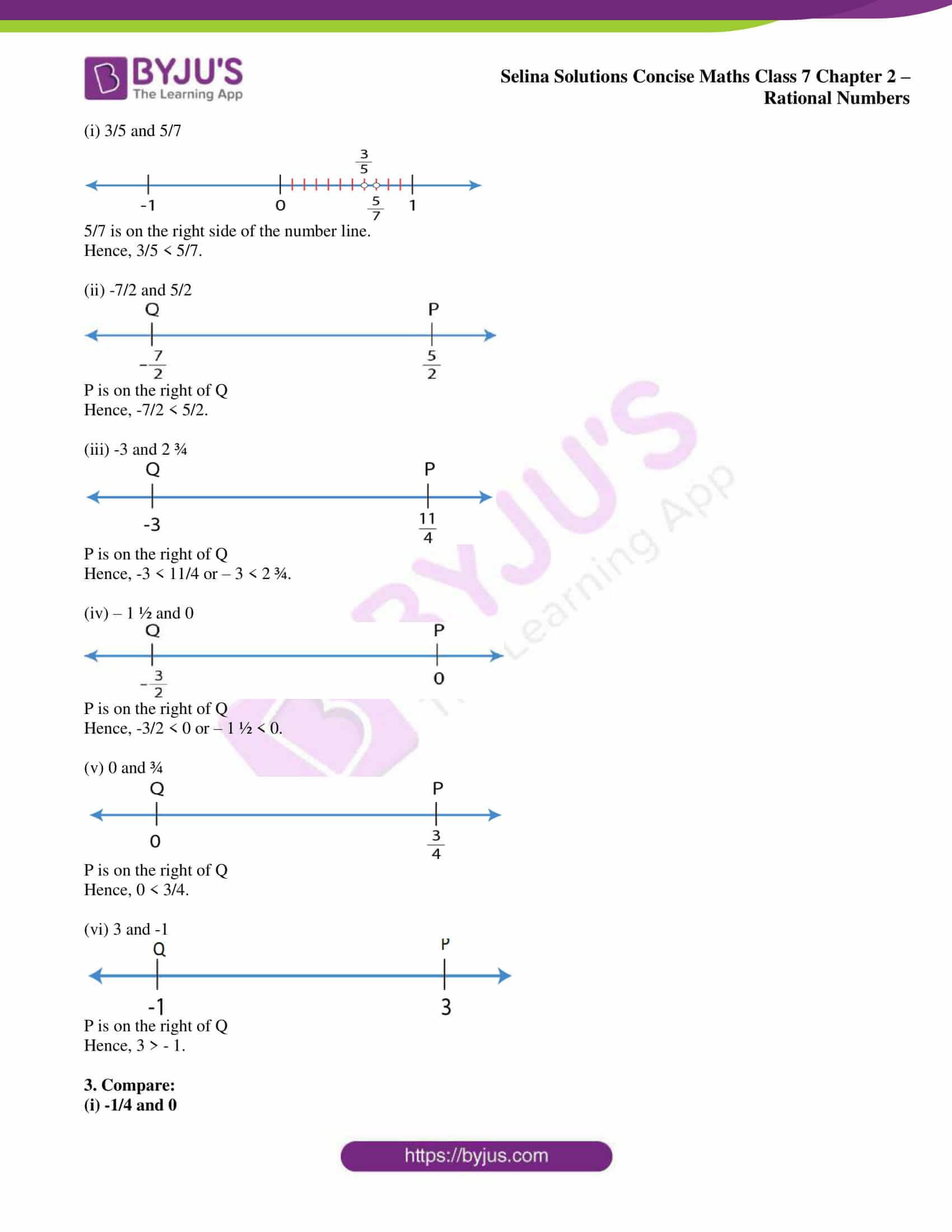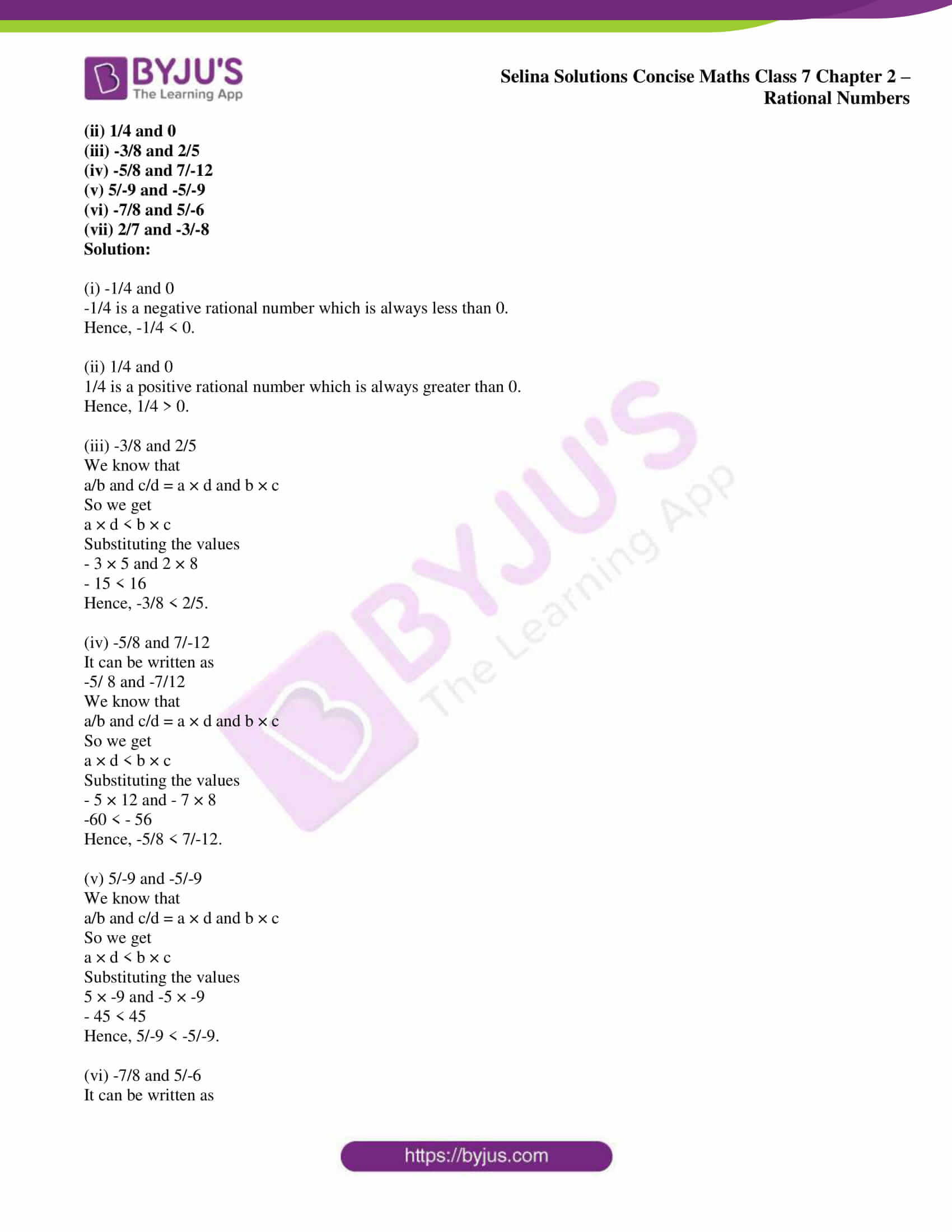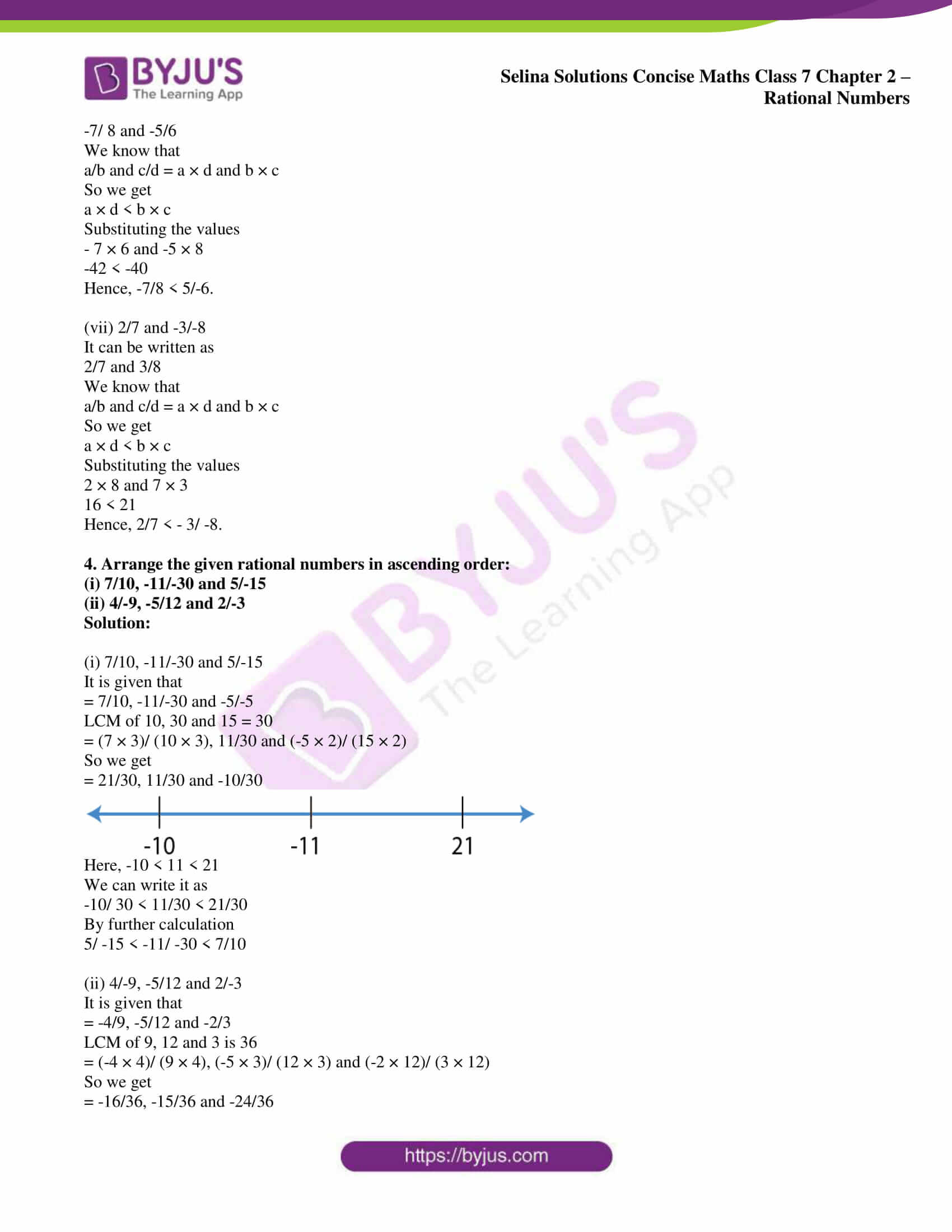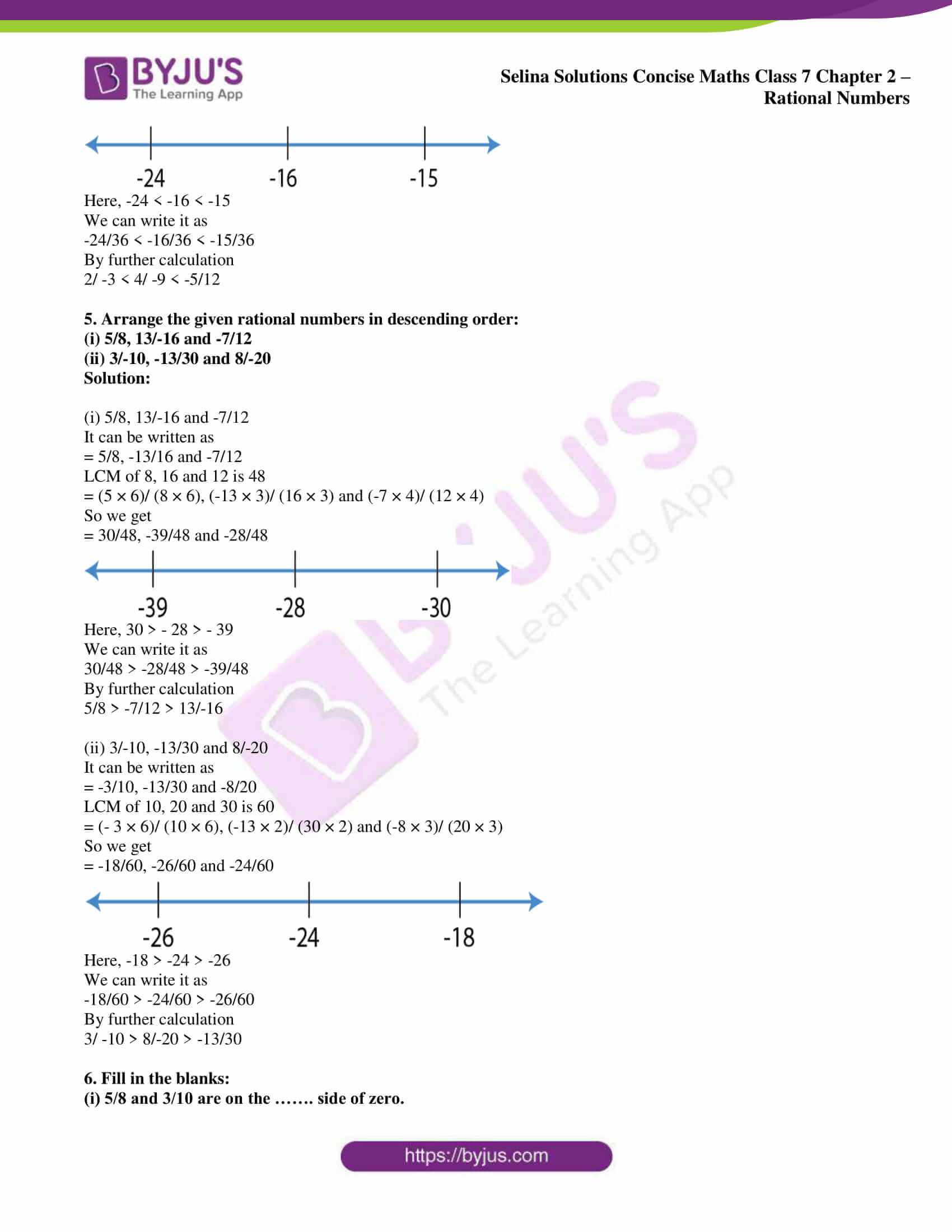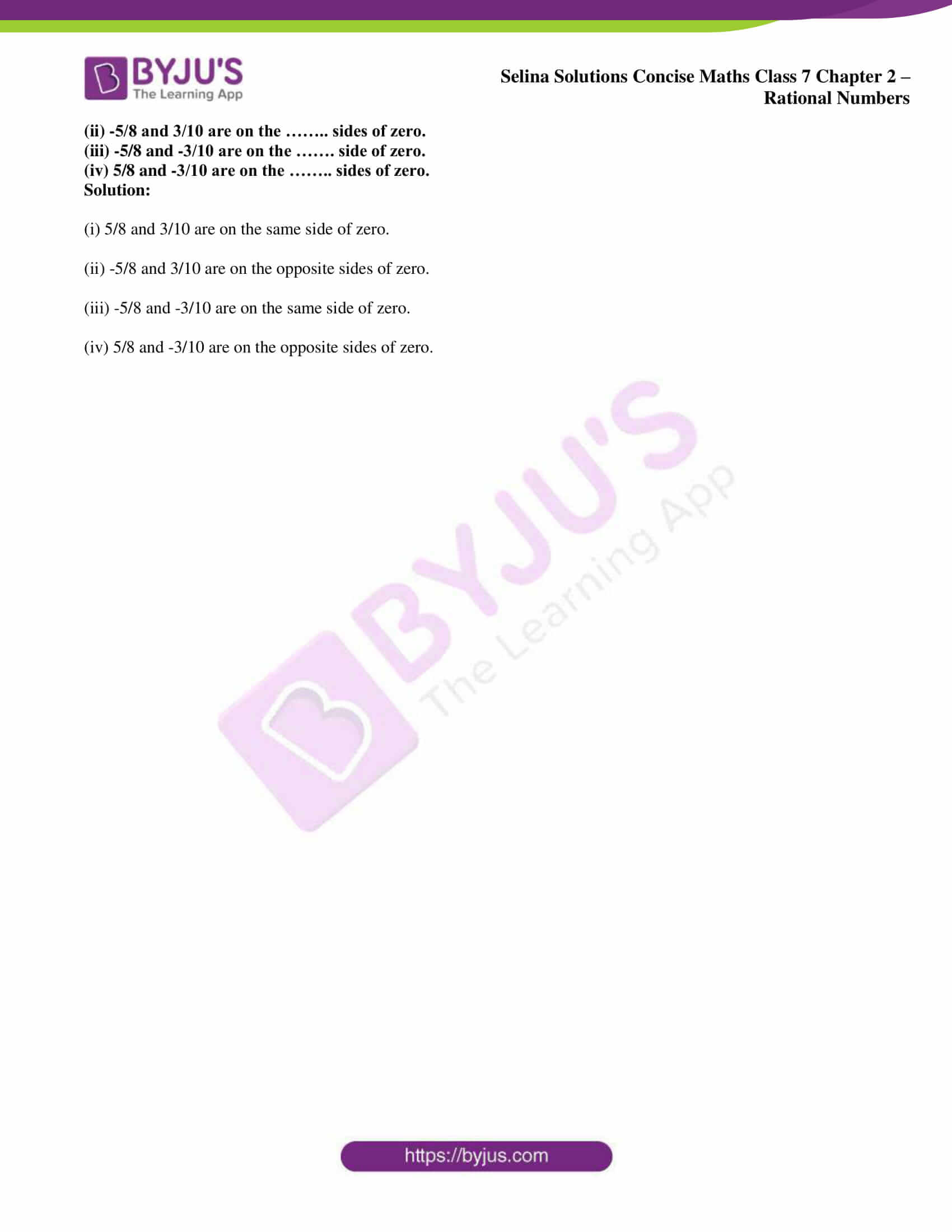### Access Selina Solutions Concise Maths Class 7 Chapter 2: Rational Numbers Exercise 2B

1. Mark the following pairs of rational numbers on the separate number lines:

(i) 3/4 and -1/4

(ii) 2/5 and -3/5

(iii) 5/6 and -2/3

(iv) 2/5 and -4/5

(v) 1/4 and -5/4

Solution:

(i) 3/4 and -1/4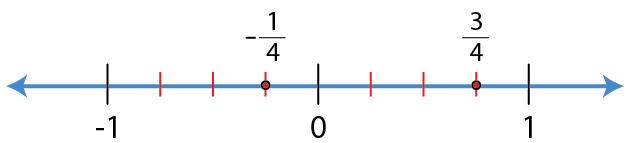(ii) 2/5 and -3/5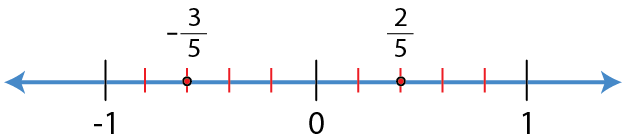(iii) 5/6 and -2/3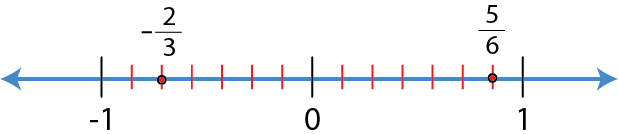(iv) 2/5 and -4/5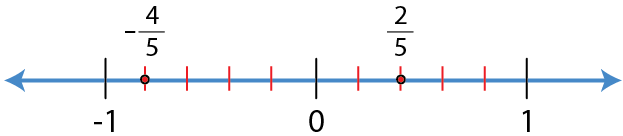(v) 1/4 and -5/4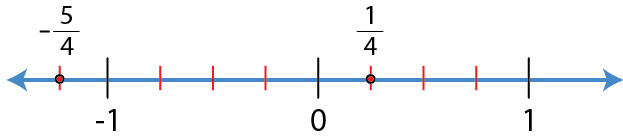2. Compare:

(i) 3/5 and 5/7

(ii) -7/2 and 5/2

(iii) -3 and 2 ¾

(iv) – 1 ½ and 0

(v) 0 and 3/4

(vi) 3 and -1

Solution:

(i) 3/5 and 5/7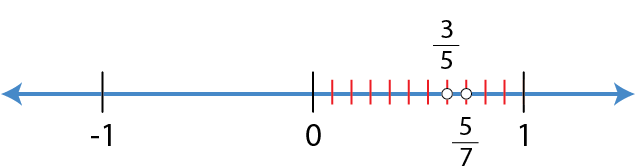5/7 is on the right side of the number line.

Hence, 3/5 < 5/7.

(ii) -7/2 and 5/2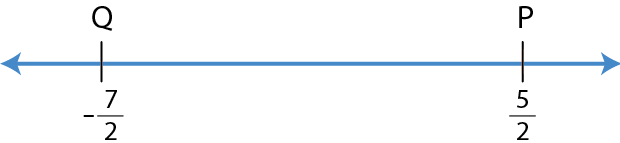P is on the right of Q

Hence, -7/2 < 5/2.

(iii) -3 and 2 ¾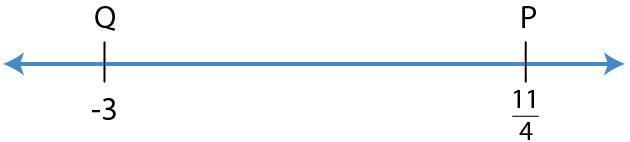P is on the right of Q

Hence, -3 < 11/4 or – 3 < 2 ¾.

(iv) – 1 ½ and 0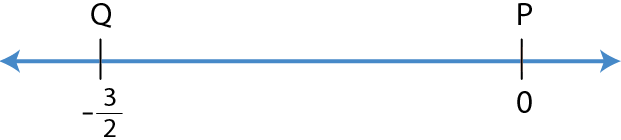P is on the right of Q

Hence, -3/2 < 0 or – 1 ½ < 0.

(v) 0 and ¾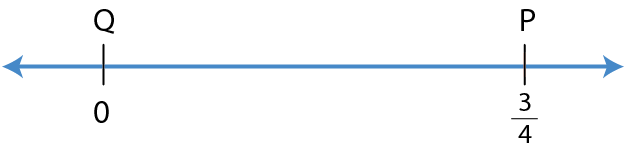P is on the right of Q

Hence, 0 < 3/4.

(vi) 3 and -1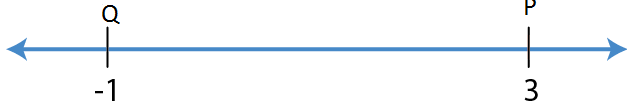P is on the right of Q

Hence, 3 > – 1.

3. Compare:

(i) -1/4 and 0

(ii) 1/4 and 0

(iii) -3/8 and 2/5

(iv) -5/8 and 7/-12

(v) 5/-9 and -5/-9

(vi) -7/8 and 5/-6

(vii) 2/7 and -3/-8

Solution:

(i) -1/4 and 0

-1/4 is a negative rational number which is always less than 0.

Hence, -1/4 < 0.

(ii) 1/4 and 0

1/4 is a positive rational number which is always greater than 0.

Hence, 1/4 > 0.

(iii) -3/8 and 2/5

We know that

a/b and c/d = a × d and b × c

So we get

a × d < b × c

Substituting the values

– 3 × 5 and 2 × 8

– 15 < 16

Hence, -3/8 < 2/5.

(iv) -5/8 and 7/-12

It can be written as

-5/ 8 and -7/12

We know that

a/b and c/d = a × d and b × c

So we get

a × d < b × c

Substituting the values

– 5 × 12 and – 7 × 8

-60 < – 56

Hence, -5/8 < 7/-12.

(v) 5/-9 and -5/-9

We know that

a/b and c/d = a × d and b × c

So we get

a × d < b × c

Substituting the values

5 × -9 and -5 × -9

– 45 < 45

Hence, 5/-9 < -5/-9.

(vi) -7/8 and 5/-6

It can be written as

-7/ 8 and -5/6

We know that

a/b and c/d = a × d and b × c

So we get

a × d < b × c

Substituting the values

– 7 × 6 and -5 × 8

-42 < -40

Hence, -7/8 < 5/-6.

(vii) 2/7 and -3/-8

It can be written as

2/7 and 3/8

We know that

a/b and c/d = a × d and b × c

So we get

a × d < b × c

Substituting the values

2 × 8 and 7 × 3

16 < 21

Hence, 2/7 < – 3/ -8.

4. Arrange the given rational numbers in ascending order:

(i) 7/10, -11/-30 and 5/-15

(ii) 4/-9, -5/12 and 2/-3

Solution:

(i) 7/10, -11/-30 and 5/-15

It is given that

= 7/10, -11/-30 and -5/-5

LCM of 10, 30 and 15 = 30

= (7 × 3)/ (10 × 3), 11/30 and (-5 × 2)/ (15 × 2)

So we get

= 21/30, 11/30 and -10/30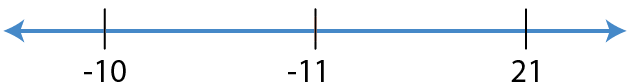Here, -10 < 11 < 21

We can write it as

-10/ 30 < 11/30 < 21/30

By further calculation

5/ -15 < -11/ -30 < 7/10

(ii) 4/-9, -5/12 and 2/-3

It is given that

= -4/9, -5/12 and -2/3

LCM of 9, 12 and 3 is 36

= (-4 × 4)/ (9 × 4), (-5 × 3)/ (12 × 3) and (-2 × 12)/ (3 × 12)

So we get

= -16/36, -15/36 and -24/36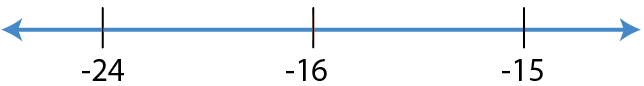Here, -24 < -16 < -15

We can write it as

-24/36 < -16/36 < -15/36

By further calculation

2/ -3 < 4/ -9 < -5/12

5. Arrange the given rational numbers in descending order:

(i) 5/8, 13/-16 and -7/12

(ii) 3/-10, -13/30 and 8/-20

Solution:

(i) 5/8, 13/-16 and -7/12

It can be written as

= 5/8, -13/16 and -7/12

LCM of 8, 16 and 12 is 48

= (5 × 6)/ (8 × 6), (-13 × 3)/ (16 × 3) and (-7 × 4)/ (12 × 4)

So we get

= 30/48, -39/48 and -28/48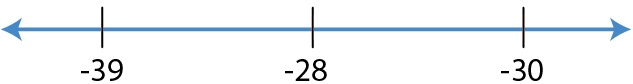Here, 30 > – 28 > – 39

We can write it as

30/48 > -28/48 > -39/48

By further calculation

5/8 > -7/12 > 13/-16

(ii) 3/-10, -13/30 and 8/-20

It can be written as

= -3/10, -13/30 and -8/20

LCM of 10, 20 and 30 is 60

= (- 3 × 6)/ (10 × 6), (-13 × 2)/ (30 × 2) and (-8 × 3)/ (20 × 3)

So we get

= -18/60, -26/60 and -24/60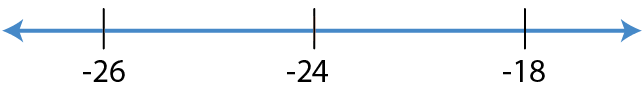Here, -18 > -24 > -26

We can write it as

-18/60 > -24/60 > -26/60

By further calculation

3/ -10 > 8/-20 > -13/30

6. Fill in the blanks:

(i) 5/8 and 3/10 are on the ……. side of zero.

(ii) -5/8 and 3/10 are on the …….. sides of zero.

(iii) -5/8 and -3/10 are on the ……. side of zero.

(iv) 5/8 and -3/10 are on the …….. sides of zero.

Solution:

(i) 5/8 and 3/10 are on the same side of zero.

(ii) -5/8 and 3/10 are on the opposite sides of zero.

(iii) -5/8 and -3/10 are on the same side of zero.

(iv) 5/8 and -3/10 are on the opposite sides of zero.